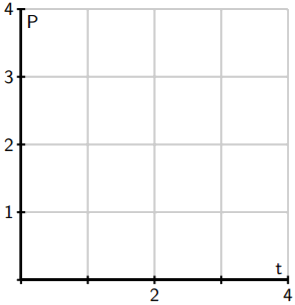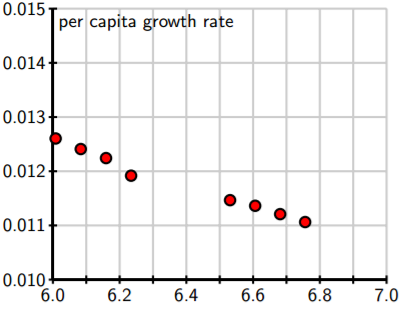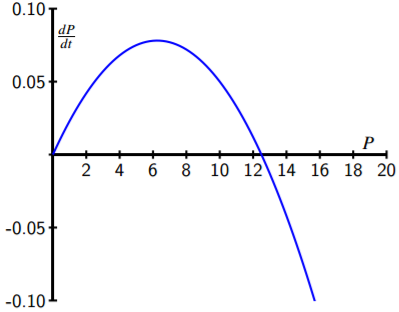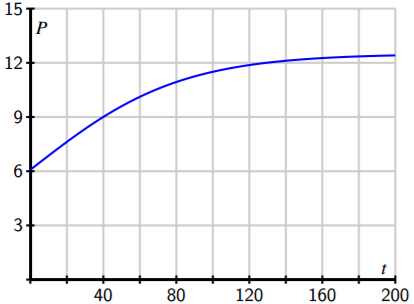$$\newcommand{\id}{\mathrm{id}}$$ $$\newcommand{\Span}{\mathrm{span}}$$ $$\newcommand{\kernel}{\mathrm{null}\,}$$ $$\newcommand{\range}{\mathrm{range}\,}$$ $$\newcommand{\RealPart}{\mathrm{Re}}$$ $$\newcommand{\ImaginaryPart}{\mathrm{Im}}$$ $$\newcommand{\Argument}{\mathrm{Arg}}$$ $$\newcommand{\norm}{\| #1 \|}$$ $$\newcommand{\inner}{\langle #1, #2 \rangle}$$ $$\newcommand{\Span}{\mathrm{span}}$$

# 7.6: Population Growth and the Logistic Equation

$$\newcommand{\vecs}{\overset { \rightharpoonup} {\mathbf{#1}} }$$ $$\newcommand{\vecd}{\overset{-\!-\!\rightharpoonup}{\vphantom{a}\smash {#1}}}$$$$\newcommand{\id}{\mathrm{id}}$$ $$\newcommand{\Span}{\mathrm{span}}$$ $$\newcommand{\kernel}{\mathrm{null}\,}$$ $$\newcommand{\range}{\mathrm{range}\,}$$ $$\newcommand{\RealPart}{\mathrm{Re}}$$ $$\newcommand{\ImaginaryPart}{\mathrm{Im}}$$ $$\newcommand{\Argument}{\mathrm{Arg}}$$ $$\newcommand{\norm}{\| #1 \|}$$ $$\newcommand{\inner}{\langle #1, #2 \rangle}$$ $$\newcommand{\Span}{\mathrm{span}}$$ $$\newcommand{\id}{\mathrm{id}}$$ $$\newcommand{\Span}{\mathrm{span}}$$ $$\newcommand{\kernel}{\mathrm{null}\,}$$ $$\newcommand{\range}{\mathrm{range}\,}$$ $$\newcommand{\RealPart}{\mathrm{Re}}$$ $$\newcommand{\ImaginaryPart}{\mathrm{Im}}$$ $$\newcommand{\Argument}{\mathrm{Arg}}$$ $$\newcommand{\norm}{\| #1 \|}$$ $$\newcommand{\inner}{\langle #1, #2 \rangle}$$ $$\newcommand{\Span}{\mathrm{span}}$$

Skills to Develop

In this section, we strive to understand the ideas generated by the following important questions:

• How can we use differential equations to realistically model the growth of a population?
• How can we assess the accuracy of our models?

The growth of the earth’s population is one of the pressing issues of our time. Will the population continue to grow? Or will it perhaps level off at some point, and if so, when? In this section, we will look at two ways in which we may use differential equations to help us address questions such as these. Before we begin, let’s consider again two important differential equations that we have seen in earlier work this chapter.

Preview Activity $$\PageIndex{1}$$

Recall that one model for population growth states that a population grows at a rate proportional to its size.

1. We begin with the differential equation
$\dfrac{dP}{dt} = \dfrac{1}{2} P. \label{1}$
Sketch a slope field below as well as a few typical solutions on the axes provided.
2. Find all equilibrium solutions of Equation $$\ref{1}$$ and classify them as stable or unstable.
3. If $$P(0)$$ is positive, describe the long-term behavior of the solution to Equation $$\ref{1}$$.
4. Let’s now consider a modified differential equation given by
$$\dfrac{dP}{dt} = \dfrac{1}{2} P(3 − P).$$
As before, sketch a slope field as well as a few typical solutions on the following axes provided.
5. Find any equilibrium solutions and classify them as stable or unstable.
6. If $$P(0)$$ is positive, describe the long-term behavior of the solution.## The Earth’s Population

We will now begin studying the earth’s population. To get started, here are some data for the earth’s population in recent years that we will use in our investigations.

 Year Population (in Billions) 1998 1999 2000 2001 2002 2005 2006 2007 2008 2009 2010 5.932 6.008 6.084 6.159 6.234 6.456 6.531 6.606 6.681 6.756 6.831

Activity $$\PageIndex{1}$$: Growth Dynamics

Our first model will be based on the following assumption:

The rate of change of the population is proportional to the population.

On the face of it, this seems pretty reasonable. When there is a relatively small number of people, there will be fewer births and deaths so the rate of change will be small. When there is a larger number of people, there will be more births and deaths so we expect a larger rate of change. If $$P(t)$$ is the population $$t$$ years after the year 2000, we may express this assumption as $\dfrac{dP}{ dt} = kP \label{eq2}$

where $$k$$ is a constant of proportionality.

1. Use the data in the table to estimate the derivative $$P'(0)$$ using a central difference. Assume that $$t = 0$$ corresponds to the year 2000.
2. What is the population $$P(0)$$?
3. Use these two facts to estimate the constant of proportionality $$k$$in the differential equation.
4. Now that we know the value of $$k$$, we have the initial value problem of Equation $$\ref{eq2}$$ with $$P(0) = 6.084$$. Find the solution to this initial value problem.
5. What does your solution predict for the population in the year 2010? Is this close to the actual population given in the table?
6. When does your solution predict that the population will reach 12 billion?
7. What does your solution predict for the population in the year 2500?
8. Do you think this is a reasonable model for the earth’s population? Why or why not? Explain your thinking using a couple of complete sentences.

Our work in Activity $$\PageIndex{1}$$ shows that that the exponential model is fairly accurate for years relatively close to 2000. However, if we go too far into the future, the model predicts increasingly large rates of change, which causes the population to grow arbitrarily large. This does not make much sense since it is unrealistic to expect that the earth would be able to support such a large population.

The constant $$k$$ in the differential equation has an important interpretation. Let’s rewrite the differential equation

$$\dfrac{dP}{dt} = kP$$

by solving for $$k$$, so that we have

$$k = \dfrac{\dfrac{dP}{dt}}{ P}.$$

Viewed in this light, $$k$$ is the ratio of the rate of change to the population; in other words, it is the contribution to the rate of change from a single person. We call this the per capita growth rate.

In the exponential model we introduced in Activity $$\PageIndex{1}$$, the per capita growth rate is constant. In particular, we are assuming that when the population is large, the per capita growth rate is the same as when the population is small. It is natural to think that the per capita growth rate should decrease when the population becomes large, since there will not be enough resources to support so many people. In other words, we expect that a more realistic model would hold if we assume that the per capita growth rate depends on the population P. In the previous activity, we computed the per capita growth rate in a single year by computing $$k$$, the quotient of $$\frac{dP}{dt}$$ and $$P$$ (which we did for $$t = 0$$). If we return data and compute the per capita growth rate over a range of years, we generate the data shown in Figure $$\PageIndex{1}$$, which shows how the per capita growth rate is a function of the population, $$P$$.Figure $$\PageIndex{1}$$: A plot of per capita growth rate vs. population P.

From the data, we see that the per capita growth rate appears to decrease as the population increases. In fact, the points seem to lie very close to a line, which is shown at two different scales in Figure $$\PageIndex{2}$$.Figure $$\PageIndex{2}$$: The line that approximates per capita growth as a function of population, P.

Looking at this line carefully, we can find its equation to be

$$\dfrac{\dfrac{dP}{dt}}{ P} = 0.025 − 0.002P.$$

If we multiply both sides by $$P$$, we arrive at the differential equation

$\dfrac{dP}{ dt} = P(0.025 − 0.002P). \label{log}$

Graphing the dependence of $$\frac{dP}{dt}$$ on the population $$P$$, we see that this differential equation demonstrates a quadratic relationship between $$\frac{dP}{dt}$$ and $$P$$, as shown in Figure $$\PageIndex{3}$$.Figure $$\PageIndex{3}$$: A plot of $$\frac{dP}{dt}$$ vs. $$P$$ for Equation $$\ref{log}$$.

Equation $$\ref{log}$$ is an example of the logistic equation, and is the second model for population growth that we will consider. We have reason to believe that it will be more realistic since the per capita growth rate is a decreasing function of the population.

Indeed, the graph in Figure $$\PageIndex{3}$$ shows that there are two equilibrium solutions, $$P = 0$$, which is unstable, and $$P = 12.5$$, which is a stable equilibrium. The graph shows that any solution with $$P(0) > 0$$ will eventually stabilize around 12.5. In other words, our model predicts the world’s population will eventually stabilize around 12.5 billion.

A prediction for the long-term behavior of the population is a valuable conclusion to draw from our differential equation. We would, however, like to answer some quantitative questions. For instance, how long will it take to reach a population of 10 billion? To determine this, we need to find an explicit solution of the equation. Solving the logistic differential equation Since we would like to apply the logistic model in more general situations, we state the logistic equation in its more general form,

$\dfrac{dP}{ dt} = kP(N − P). \label{7.2}$

The equilibrium solutions here are when $$P = 0$$ and $$1 − \frac{P}{N} = 0$$, which shows that $$P = N$$. The equilibrium at $$P = N$$ is called the carrying capacity of the population for it represents the stable population that can be sustained by the environment.

We now solve the logistic Equation $$\ref{7.2}$$, which is separable, so we separate the variables

$$\dfrac{1}{P(N − P)} \dfrac{ dP}{ dt} = k,$$

and integrate to find that

$$\int \dfrac{1}{P(N − P)} dP = \int k dt,$$

To find the antiderivative on the left, we use the partial fraction decomposition

$$\dfrac{1}{P(N − P)} = \dfrac{1}{ N} \left[ \dfrac{ 1}{ P} + \dfrac{1}{ N − P} \right] .$$

Now we are ready to integrate, with

$$\int \dfrac{1}{ N} \left[ \dfrac{1}{ P} + \dfrac{1}{ N − P} \right] dP = \int k dt.$$

On the left, observe that $$N$$ is constant, so we can remove the factor of $$\frac{1}{N}$$ and antidifferentiate to find that

$$\dfrac{1}{ N} (\ln |P| − \ln |N − P|) = kt + C.$$

Multiplying both sides of this last equation by $$N$$ and using an important rule of logarithms, we next find that

$$\ln \left| \dfrac{P}{ N − P} \right | = kNt + C.$$

From the definition of the logarithm, replacing $$e^C$$ with $$C$$, and letting $$C$$ absorb the absolute value signs, we now know that

$$\dfrac{P}{N − P} = Ce^{k N t}.$$

At this point, all that remains is to determine $$C$$ and solve algebraically for $$P$$.

If the initial population is $$P(0) = P_0$$, then it follows that

$$C = \dfrac{P_0}{ N−P_0}$$

so

$$\dfrac{P}{N − P} = \dfrac{P_0}{ N − P_0} e^{ k N t} .$$

We will solve this most recent equation for $$P$$ by multiplying both sides by $$(N − P)(N − P_0)$$ to obtain

\begin{align} P(N − P_0) & = P_0(N − P)e^{k N t} \\ & = P_0Ne^{k N t} − P_0Pe^{k N t}. \end{align}

Swapping the left and right sides, expanding, and factoring, it follows that

\begin{align} P_0Ne^{k N t} & = P(N − P_0) + P_0Pe^{k N t} \\ & = P(N − P_0 + P_0e^{ k N t}). \end{align}

Dividing to solve for $$P$$, we see that

$$P = \dfrac{P_0Ne^{k N t}}{ N − P_0 + P_0e^{k N t}}.$$

Finally, we choose to multiply the numerator and denominator by $$\frac{1}{P_0} e^{−k N t}$$ to obtain

$P(t) = \dfrac{N}{ \left( \dfrac{N−P_0}{P_0} \right) e^{−k N t} + 1} . \label{7.3}$

While that was a lot of algebra, notice the result: we have found an explicit solution to the initial value problem

$$\dfrac{dP}{dt} = kP(N − P),\ P(0)=P_{0},$$

with $$P(0) = P_0$$ and that solution is Equation $$\ref{7.3}$$.

For the logistic equation describing the earth’s population that we worked with earlier in this section, we have

$$k = 0.002$$, $$N = 12.5$$, and $$P_0 = 6.084$$.

This gives the solution

$P(t) = \dfrac{12.5}{ 1.0546e^{−0.025t} + 1}, \label{earth}$

whose graph is shown in Figure $$\PageIndex{4}$$ Notice that the graph shows the population leveling off at 12.5 billion, as we expected, and that the population will be around 10 billion in the year 2050. These results, which we have found using a relatively simple mathematical model, agree fairly well with predictions made using a much more sophisticated model developed by the United Nations.Figure $$\PageIndex{4}$$: The solution to the logistic equation modeling the earth’s population (Equation \ref{earth}).

The logistic equation is useful in other situations, too, as it is good for modeling any situation in which limited growth is possible. For instance, it could model the spread of a flu virus through a population contained on a cruise ship, the rate at which a rumor spreads within a small town, or the behavior of an animal population on an island. Again, it is important to realize that through our work in this section, we have completely solved the logistic equation, regardless of the values of the constants $$N$$, $$k$$, and $$P_0$$.

Anytime we encounter a logistic equation, we can apply the formula we found in Equation \ref{7.3}.

Activity $$\PageIndex{2}$$: Predicting Earth's Population

Consider the logistic equation

$$\dfrac{dP}{dt} = kP(N − P) \nonumber$$

with the graph of $$\frac{dP}{dt}$$ vs. $$P$$ shown below.1. At what value of $$P$$ is the rate of change greatest?
2. Consider the model for the earth’s population that we created. At what value of $$P$$ is the rate of change greatest? How does that compare to the population in recent years?
3. According to the model we developed, what will the population be in the year 2100?
4. According to the model we developed, when will the population reach 9 billion?
5. Now consider the general solution to the general logistic initial value problem that we found, given by Equation $$\ref{7.3}$$ . Verify algebraically that $$P(0) = P_0$$ and that $$\lim_{t→\infty} P(t) = N.$$

## Summary

In this section, we encountered the following important ideas:

• If we assume that the rate of growth of a population is proportional to the population, we are led to a model in which the population grows without bound and at a rate that grows without bound.
• By assuming that the per capita growth rate decreases as the population grows, we are led to the logistic model of population growth, which predicts that the population will eventually stabilize at the carrying capacity.

## Contributors

Matt Boelkins (Grand Valley State University), David Austin (Grand Valley State University), Steve Schlicker (Grand Valley State University)